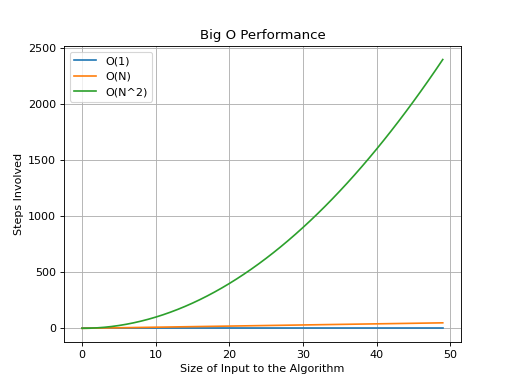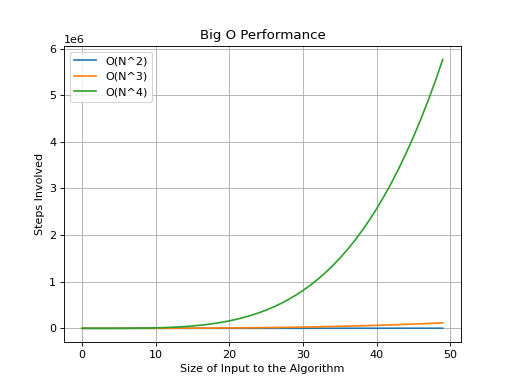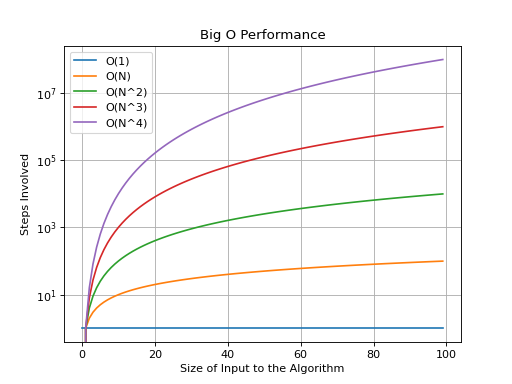# Data Structures and Algorithms¶

This repository will hold my Python implementations of various data structures and algorithms.

## Resources¶

1. MIT 6.006 Introduction to Algorithms

2. Problem Solving with Algorithms and Data Structures using Python

3. Grokking Algorithms

4. MIT 6.046 Design and Analysis of Algorithms

## Big O Performance Charts¶

These charts hope to show just how much each of the Big O categories vary with respect to each other.

The first chart shows the performance of $$O(1)$$ and $$O(N)$$ with respect to $$O(N^2)$$.This next chart shows the performance of $$O(N^2)$$ and $$O(N^3)$$ with respect to $$O(N^4)$$.Let’s look at these with a logarithmic Y-axis.These graphs go on to show just how large the difference is between each of the categories.